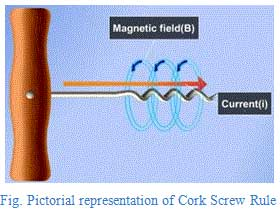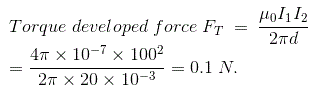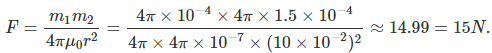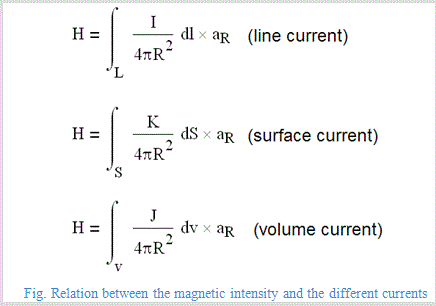# MCQs on Electromagnetic Fields

##### Page 2 of 20. Go to page 1 2 3 4 5 6 7 8 9 10 11 12 13 14 15 16 17 18 19 20
01․ The force between two long parallel conductors is inversely proportional to
current in one conductor.
distance between the conductors.
product of current in two conductors.

If the current is flowing through the two different conductors ‘d’ distance apart, in the same direction, then the force between the two conductors is directly proportional to the product of the currents and inversely proportional to the distance between the two conductors.

02․ In the left hand rule, forefinger always represents
voltage.
current.
magnetic field.
direction of force on the conductor.Thumb finger represents the direction of force, Fore finger represents the direction of magnetic field, middle finger represents the direction of current.

03․ A wire carrying current is bent in the form of a circular loop. Then the magnetic field around each portion of the wire will be
parallel to the plane of the wire.
perpendicular to the circumference of the wire.
parallel to half portion and perpendicular for the other half.
none of above.

Suppose the straight wire is bent in the form of a circular loop, current flows through every point on the wire that would give rise to the magnetic field appearing as straight lines at the center of the circular loop. By applying the right hand rule, it is easy to check that every section of the wire contributes to the magnetic field lines in the same direction within the loop.

04․ Cork Screw rule is used to find
direction of current.
direction of magnetic field.
direction of electric field.
direction of emf.

The direction in which the head of the cork screw rotates that would give the direction of the magnetic field or magnetic force of lines. The shape of this magnetic field is circular and the direction of magnetic field is clock-wise.05․ Two long parallel conductors carry 100 A. If the conductors are separated by 20 mm, the force per meter of length of each conductor will be
100 N.
0.1 N.
1 N.
0.01 N.

Current I1 and I2 = 100 Amps, Distance = 20 mm = 20 × 10-3 m, Force = ? The force is found by the following expression06․ The field at any point on the axis of a current carrying coil will be
at angle of 45° with the axis.
perpendicular to the axis.
parallel to axis.
zero.

A continuous current in a current carrying coil is divided into multiple current elements. Using the superposition principle and the Biot-Savarts law, each discrete element generates its own magnetic field and when it is integrated with each field that produces a resultant field and it is aligned parallel to the axis of the coil.

07․ Two straight parallel conductors carry equal currents in opposite direction. The force between them is
repulsive.
attractive.
zero.
none of above.

When two current carrying conductors are placed parallelly, there will be a force acting between them. The force is attractive if direction of currents in the conductor is same and it is repulsive if the direction of the currents is opposite. This can easily be explained by right hand rule.

08․ The change of cross sectional area of conductor in magnetic field will affect
reluctance of conductor.
resistance of conductor.
(A) and (B) both in the same way.
none of above.

We know that the cross sectional area is inversely proportional to the resistance of the conductor. In magnetic circuits, reluctance is analogous to the resistance. Hence, the resistance and reluctance would be affected in the same way.

09․ A point pole has a strength of 4π × 10-4 weber. The force in newtons on a point pole of 4π × 1.5 × 10-4 weber placed at a distance of 10 cm from it will be
15 N.
20 N.
7.5 N.
3.75 N.

Given data: Pole strength m1 = 4π × 10-7 Weber m2 = 4π × 1.5 × 10-4; r = 10 cm. We know that the force is found by the below expression,10․ Biot-Savarts law states the relation between magnetic intensity and
filament current only.
surface current only.
(A), (B), and (D) are all correct.
volume current only.

Biot-Savarts law states that the differential magnetic field intensity dH produced at a point P by the differential current element Idl. This is the only one law is available to relate the magnetic intensity and the different type of current distribution. The given equations show the different relations between the magnetic intensity and the currents.<<<1234>>>